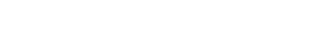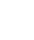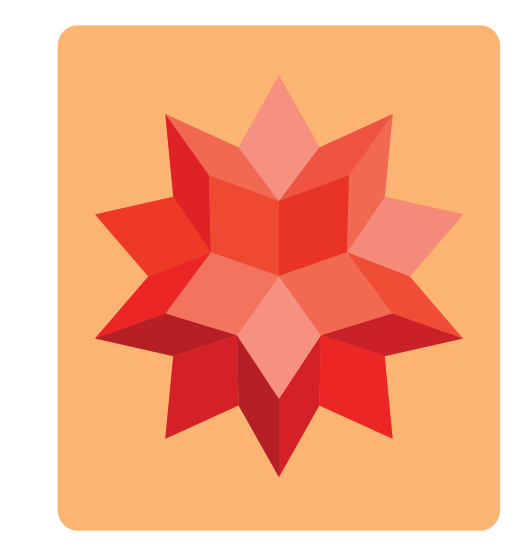#Wolfram community resources and data for COVID-19 research     Resources for COVID-19 research and outreach for remote learning and workThe simplicity of Wolfram|Alpha with the computational capabilities of Mathematica.

Try Now Pricing#Wolfram|Alpha Notebook Edition combines the best of both Wolfram|Alpha and Mathematica into a single, unified tool perfect for teaching and learning. Use free-form input to get instant answers to questions, create and customize graphs, and turn static examples into dynamic models. Everything is saved as an interactive Wolfram Notebook, so you can add notes and use notebooks as class or reference materials, or present them as dynamic slide shows that engage your audience as you edit examples on the fly.

## Intuitive and easy to use### Free-form input

Enter plain English and get instant answers using Wolfram|Alpha technology.

### Suggestions for next steps

Finish a computation and get personalized suggestions about what to do next.

### Free-form input

Enter plain English and get instant answers using Wolfram|Alpha technology.

### Suggestions for next steps

Finish a computation and get personalized suggestions about what to do next.

## Understand difficult math and science concepts### Use real-world data

Utilize up-to-date data from Wolfram|Alpha to make your calculations real-world relevant.

### Step-by-step math equations

Learn how to solve math problems with a self-assessment tutor, featuring steps and hints that guide you from problems to answers.

### Build up calculations

Use previous results in future calculations, assign variables and create your own functions.

### Create interactive coursework

Develop lessons, quizzes and assignments that students can explore on their own.

### Use real-world data

Utilize up-to-date data from Wolfram|Alpha to make your calculations real-world relevant.

### Step-by-step math equations

Learn how to solve math problems with a self-assessment tutor, featuring steps and hints that guide you from problems to answers.

### Build up calculations

Use previous results in future calculations, assign variables and create your own functions.

### Create interactive coursework

Develop lessons, quizzes and assignments that students can explore on their own.

## Calculate, document, present—in one unified program### Customize notes, quizzes and papers

Personalize your documents with titles, sections, lists, citations and fully typeset mathematical notation.

### Interactive manipulates

Quickly turn static examples into dynamic models to visualize complex concepts easily.

### All-in-one environment

Combine calculations, graphics, interactive examples and notes in a single document.

### Dynamic slide shows

Turn your notebook into a slide show presentation—complete with interactive elements that can be edited on the fly.

### Customize notes, quizzes and papers

Personalize your documents with titles, sections, lists, citations and fully typeset mathematical notation.

### Interactive manipulates

Quickly turn static examples into dynamic models to visualize complex concepts easily.

### All-in-one environment

Combine calculations, graphics, interactive examples and notes in a single document.

### Dynamic slide shows

Turn your notebook into a slide show presentation—complete with interactive elements that can be edited on the fly.